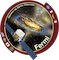### Source Report Table Description

 Date: Mid point of time bin in UTC. Julian date: Mid point of time bin in JD. MET: Mid point of time bin in Mission Elapsed Time (MET). TS: The likelihood Test Statistic. Photon Flux: Average integrated photon flux with energies from 0.1 to 100 GeV. Units in photons/cm^2/s. Photon Flux Error: 1-sigma symmetric gaussian equivalent statistical uncertainty based on the inverse-Hessian at the optimum of the log-likelihood surface. Units in photons/cm^2/s. Energy Flux: Average integrated energy flux with energies from 0.1 to 100 GeV. Units in MeV/cm^2/s. Energy Flux Error: 1-sigma symmetric gaussian equivalent statistical uncertainty based on the inverse-Hessian at the optimum of the log-likelihood surface. Units in MeV/cm^2/s. Photon Index: Average photon index, if the spectral model for the source in the FGL catalog used by the LCR is a power-law. Average alpha, if the spectral model for the source in the FGL catalog used by the LCR is a logparabola. Photon Index Error: 1-sigma symmetric gaussian equivalent photon index error Fit Tolerance: Relative fit tolerance. MINUIT Return Code: Anything other than '0' means that the analysis did not converge. Please read the caveats about non-convergent analyses. Analysis Log: Complete LAT analysis runtime output of data within the time invertal of the bin.

### JSON File Description

Some of the objects below are provided as arrays of 2-tuples where the first element is the center of the bin time interval (in MET).
 ts: The likelihood Test Statistic. flux: Average integrated flux of photons with energies from 0.1 to 100 MeV. Units in photons/cm^2/s if photon flux is selected, MeV/cm^2/s if energy flux is selected. flux_upper_limits: 95% Flux upper limits in photons/cm^2/s. flux_error: 1-sigma symmetric gaussian equivalent statistical uncertainty in photons/cm^2/s if photon flux is selected, MeV/cm^2/s if energy flux is selected. photon_index: Photon index if source spectral shape is power-law, alpha if it is logparabola. photon_index_error: 1-sigma symmetric gaussian equivalent photon index/alpha statistical uncertainty. fit_tolerance: Relative fit tolerance. fit_convergence: MINUIT return code. Anything other than '0' means that the analysis did not converge. Please read the caveats about non-convergent analyses. dlogl: Delta log-likelihood between a fixed index and free index fit. EG: Isotropic diffuse background Normalization. GAL: Galactic diffuse background Prefactor. bin_id: Time bin number.